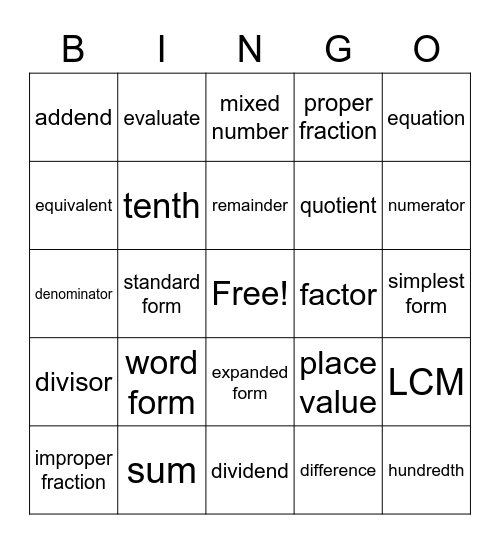# Math VocabularyThis bingo card has a free space and 29 words: improper fraction, decimal, standard form, product, addend, mixed number, tenth, word form, factor, sum, simplest form, hundredth, dividend, difference, numerator, thousandth, expanded form, divisor, equation, denominator, place value, ones, quotient, equivalent, proper fraction, evaluate, LCM, remainder and GCF.

## Play Online